# 非线性降维方法 Isomap Embedding

2021/08/30 08:50

Isomap Embedding 等距特征映射是一种新颖,高效的非线性降维技术,它的一个突出优点是只有两个参数需要设定,即邻域参数和嵌入维数.

1. Isomap 属于哪一类机器学习技术？

2. Isomap 是如何工作的？我通过一个直观的例子而不是复杂的数学来解释。

3. 如何使用 Isomap 减少数据的维度？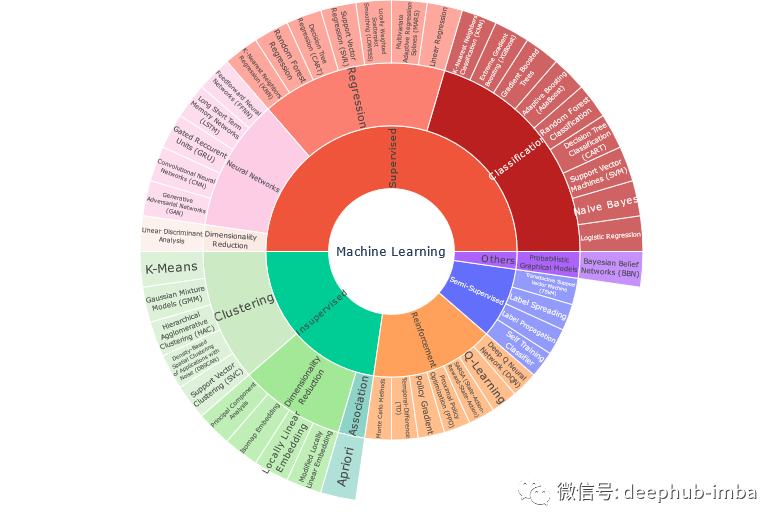## 等距映射 (Isomap) 如何工作？

Isomap 是一种结合了几种不同算法的技术，使其能够使用非线性方式来减少维度，同时保留局部结构。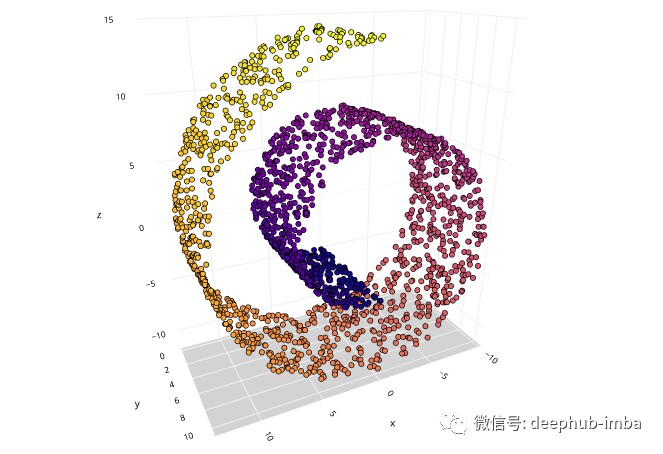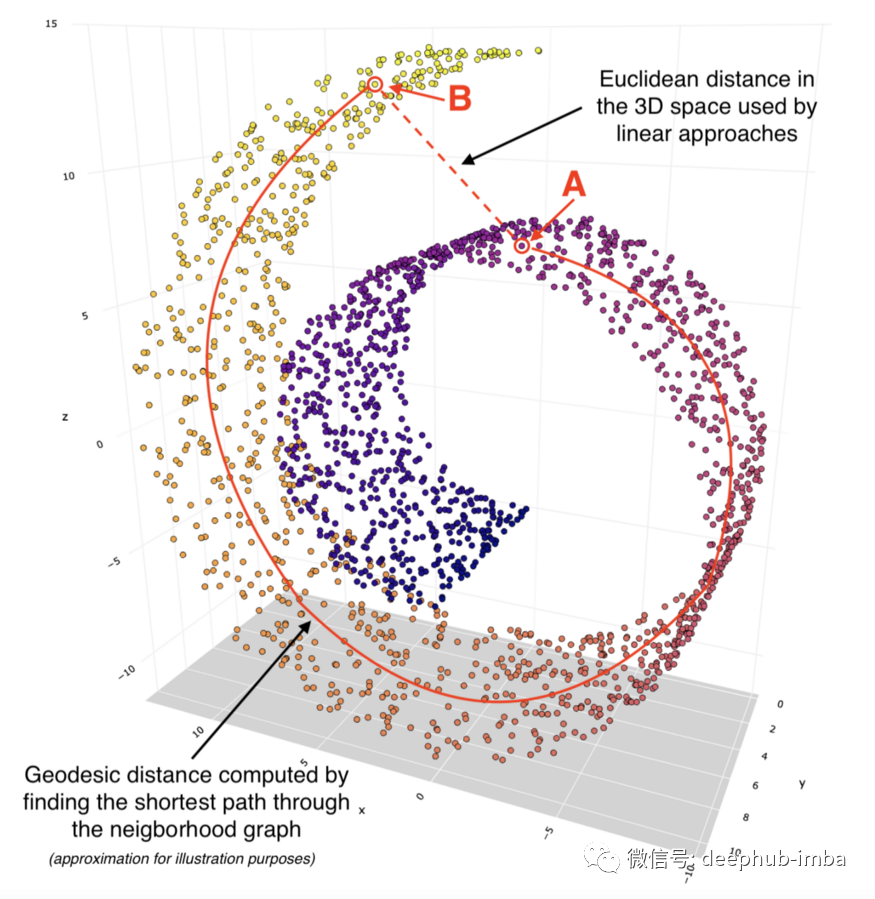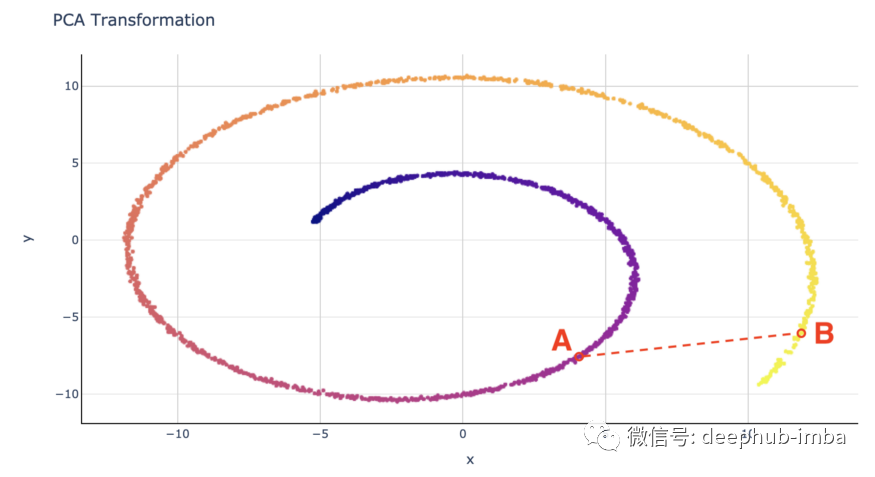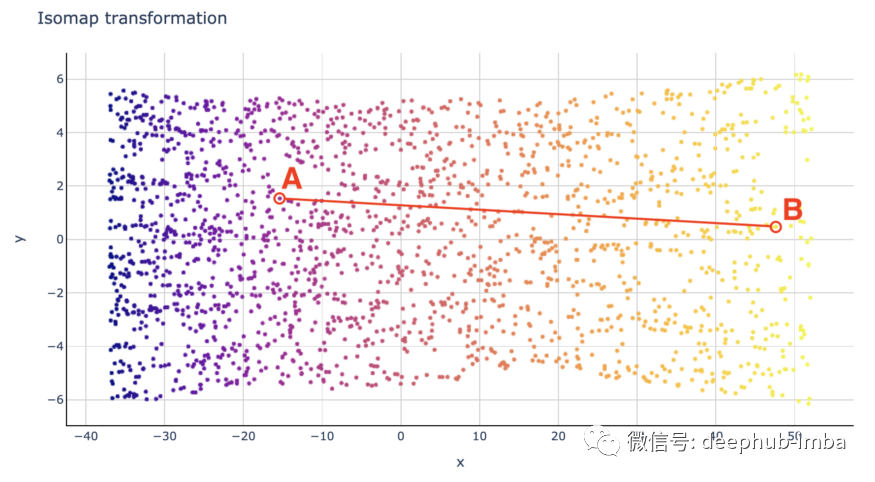## 如何使用 Isomap ？

• Scikit-learn

• Plotly 和 Matplotlib

• Pandas

 import pandas as pd # for data manipulation  # Visualization import plotly.express as px # for data visualization import matplotlib.pyplot as plt # for showing handwritten digits  # Skleran from sklearn.datasets import load_digits # for MNIST data from sklearn.manifold import Isomap # for Isomap dimensionality reduction

 # Load digits data  digits = load_digits()  # Load arrays containing digit data (64 pixels per image) and their true labels X, y = load_digits(return_X_y=True)  # Some stats print('Shape of digit images: ', digits.images.shape) print('Shape of X (training data): ', X.shape) print('Shape of y (true labels): ', y.shape)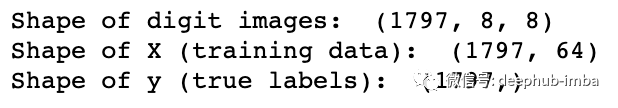# Display images of the first 10 digits fig, axs = plt.subplots(2, 5, sharey=False, tight_layout=True, figsize=(12,6), facecolor='white') n=0 plt.gray()  for i in range(0,2):     for j in range(0,5):         axs[i,j].matshow(digits.images[n])         axs[i,j].set(title=y[n])         n=n+1 plt.show()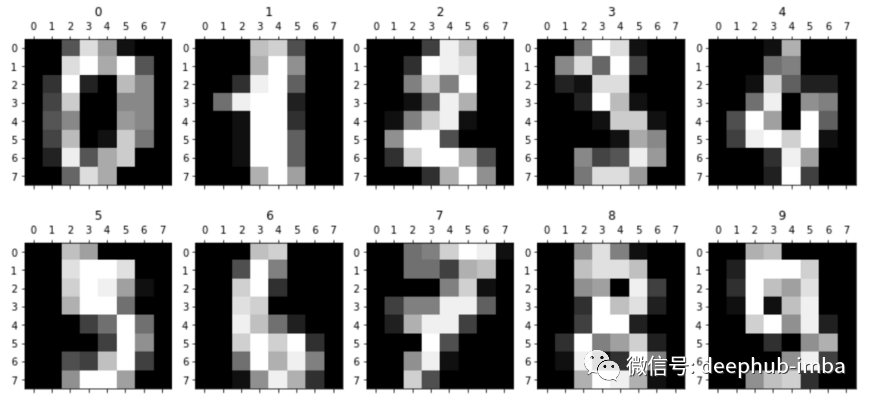### Step 1 - Configure the Isomap function, note we use default hyperparameter values in this example embed3 = Isomap(     n_neighbors=5, # default=5, algorithm finds local structures based on the nearest neighbors     n_components=3, # number of dimensions     eigen_solver='auto', # {‘auto’, ‘arpack’, ‘dense’}, default=’auto’     tol=0, # default=0, Convergence tolerance passed to arpack or lobpcg. not used if eigen_solver == ‘dense’.     max_iter=None, # default=None, Maximum number of iterations for the arpack solver. not used if eigen_solver == ‘dense’.     path_method='auto', # {‘auto’, ‘FW’, ‘D’}, default=’auto’, Method to use in finding shortest path.     neighbors_algorithm='auto', # neighbors_algorithm{‘auto’, ‘brute’, ‘kd_tree’, ‘ball_tree’}, default=’auto’     n_jobs=-1, # n_jobsint or None, default=None, The number of parallel jobs to run. -1 means using all processors     metric='minkowski', # string, or callable, default=”minkowski”     p=2, # default=2, Parameter for the Minkowski metric. When p = 1, this is equivalent to using manhattan_distance (l1), and euclidean_distance (l2) for p = 2     metric_params=None # default=None, Additional keyword arguments for the metric function. )  ### Step 2 - Fit the data and transform it, so we have 3 dimensions instead of 64 X_trans3 = embed3.fit_transform(X)      ### Step 3 - Print shape to test print('The new shape of X: ',X_trans3.shape)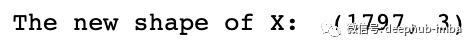# Create a 3D scatter plot fig = px.scatter_3d(None,                      x=X_trans3[:,0], y=X_trans3[:,1], z=X_trans3[:,2],                     color=y.astype(str),                     height=900, width=900                    )  # Update chart looks fig.update_layout(#title_text="Scatter 3D Plot",                   showlegend=True,                   legend=dict(orientation="h", yanchor="top", y=0, xanchor="center", x=0.5),                   scene_camera=dict(up=dict(x=0, y=0, z=1),                                          center=dict(x=0, y=0, z=-0.2),                                         eye=dict(x=-1.5, y=1.5, z=0.5)),                                         margin=dict(l=0, r=0, b=0, t=0),                   scene = dict(xaxis=dict(backgroundcolor='white',                                           color='black',                                           gridcolor='#f0f0f0',                                           title_font=dict(size=10),                                           tickfont=dict(size=10),                                          ),                                yaxis=dict(backgroundcolor='white',                                           color='black',                                           gridcolor='#f0f0f0',                                           title_font=dict(size=10),                                           tickfont=dict(size=10),                                           ),                                zaxis=dict(backgroundcolor='lightgrey',                                           color='black',                                            gridcolor='#f0f0f0',                                           title_font=dict(size=10),                                           tickfont=dict(size=10),                                          )))  # Update marker size fig.update_traces(marker=dict(size=2))  fig.show()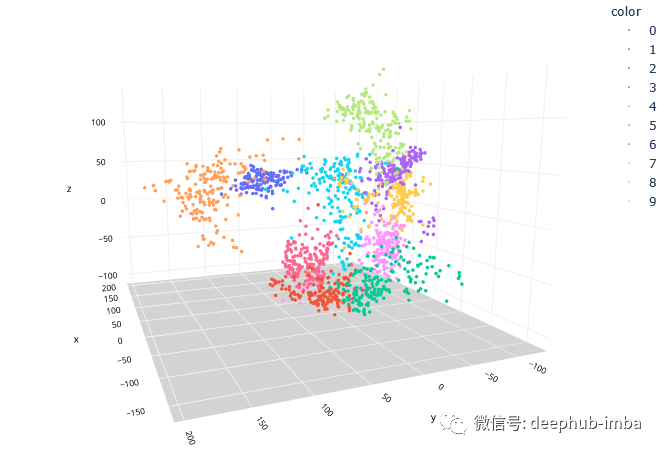Isomap 在将维度从 64 减少到 3 方面做得非常出色，同时保留了非线性关系。这使我们能够在 3 维空间中可视化手写数字的簇。

## 总结

Isomap 是降维的最佳工具之一，使我们能够保留数据点之间的非线性关系。0 评论
0 收藏
0